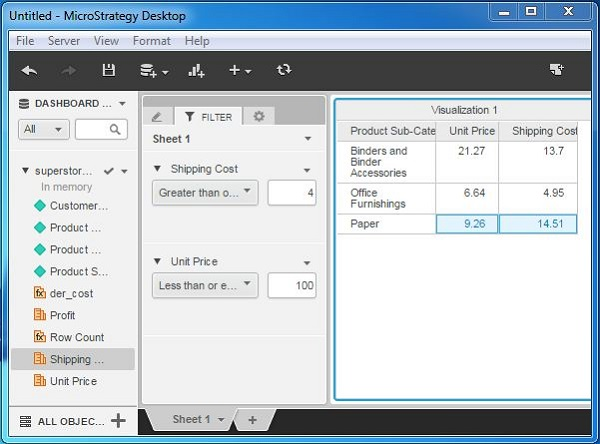# MicroStrategy - Metric Comparison

Metrics are the numerical values on which we can apply mathematical calculations and also compare them numerically. MicroStrategy desktop provides some functionality to compare the values of two metrics using the filtering functions. If required, we can also create a derived metric to make complex comparisons based on some specific calculation.

Following are the steps to create a comparison between two metrics.

## Step 1

Create a visualization with the grid report using the superstore.xlx as an example data set. Next, drag the two metrics - Unit price and Shipping cost - under the filter tab as shown in the following screenshot.## Step 2

Enter some specific values in the filter condition of both the metrics, so that we can compare their values within a range. The following screenshot shows the result after entering the values.